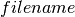NetworkX

Exceptions

is_string_like

# Utilities¶

## Helper functions¶

Miscellaneous Helpers for NetworkX.

These are not imported into the base networkx namespace but can be accessed, for example, as

```>>> import networkx
>>> networkx.utils.is_string_like('spam')
True
```
 is_string_like(obj) Check if obj is string. flatten(obj[, result]) Return flattened version of (possibly nested) iterable object. iterable(obj) Return True if obj is iterable with a well-defined len(). is_list_of_ints(intlist) Return True if list is a list of ints. make_str(t) Return the string representation of t. cumulative_sum(numbers) Yield cumulative sum of numbers. generate_unique_node() Generate a unique node label. default_opener(filename) Opensusing system’s default program.

## Data structures and Algorithms¶

Union-find data structure.

 UnionFind.union(*objects) Find the sets containing the objects and merge them all.

## Random sequence generators¶

Utilities for generating random numbers, random sequences, and random selections.

 create_degree_sequence(n[, sfunction, max_tries]) Attempt to create a valid degree sequence of length n using specified function sfunction(n,**kwds). pareto_sequence(n[, exponent]) Return sample sequence of length n from a Pareto distribution. powerlaw_sequence(n[, exponent]) Return sample sequence of length n from a power law distribution. uniform_sequence(n) Return sample sequence of length n from a uniform distribution. cumulative_distribution(distribution) Return normalized cumulative distribution from discrete distribution. discrete_sequence(n[, distribution, ...]) Return sample sequence of length n from a given discrete distribution or discrete cumulative distribution. zipf_sequence(n[, alpha, xmin]) Return a sample sequence of length n from a Zipf distribution with zipf_rv(alpha[, xmin, seed]) Return a random value chosen from the Zipf distribution. random_weighted_sample(mapping, k) Return k items without replacement from a weighted sample. weighted_choice(mapping) Return a single element from a weighted sample.

## Decorators¶

 open_file(path_arg[, mode]) Decorator to ensure clean opening and closing of files. require(*packages) Decorator to check whether specific packages can be imported.